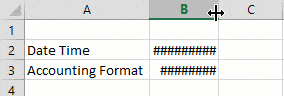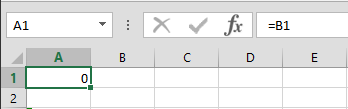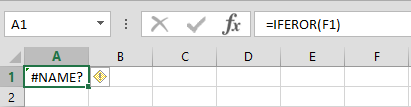Troubleshooting issues in Excel can be easier than you might think, if you know how to interpret error messages. Error messages almost always start with a hashtag, followed by the error code. We’ve prepared this guide to help you understand the way errors are handled in Excel and suggest some workarounds or solutions as to how you can get rid of them. Press the button below to download our sample workbook that contains all errors we’re going to cover. Let’s take a look at 8 most common formula errors together.

## 1.    #####

Not a real error, but rather an inconvenience, Excel shows a set of hashtags when the value displayed on a cell doesn’t fit into the visible area. This occurs more often when working with dates, as full date and time text usually won’t fit into the regular size cells. There really is no way to get rid of this, aside from increasing the width of the column!## 2.    #VALUE!

Excel will give you a #VALUE! error when an argument in your formula that is supposed to be a number, contains text or special characters. For example, adding a text with numbers can cause this error.Best workaround for this is to double check your formula and avoid using non-numeric values where possible. If you have to use non-numeric values, you can use functions that handle non-numeric values automatically, such as SUM or IF.

## 3.    #DIV/0!

Math can’t calculate division by 0, and neither can Excel. #DIV/0 error is displayed when a formula is trying to divide a number by the number 0. Please note that empty cells are processed as zeros in Excel’s formulas.Begin by double checking your formula and make sure all parameters are entered correctly. If, for some reason, zero values and the subsequent errors are inevitable, you can use the IFERROR function to bypass this error.

## 4.    #REF!

You will see the #REF! error when there is an invalid reference (a cell, a range or a table). This could mean that the reference was removed and Excel can’t find it. Deleting a column, a row or a sheet completely are possible causes.The only viable workaround is to bring back the original reference.## 5.    #N/A!

You will see the #N/A! error when a formula can’t find the reference value. This error is pretty common when searching for a value using VLOOKUP, in a table that doesn’t contain the lookup value.To find the root cause, double check both your formula, and the reference range you are working in.

## 6.    #NAME?

The #NAME? error occurs when Excel can’t recognize a formula, a name, or a reference. This error typically occurs because of a typo or a missing named range. For example, entering IFEROR instead of IFERROR will display this message.Using a named range that wasn’t defined in the workbook will give the same error.Check your formula for typos and make sure that all named ranges are defined in your workbook.

## 7.    #NUM!

Excel will give the #NUM! error if it encounters an invalid number within a formula. Common example are,

## 8.    #NULL!

You will get the #NULL! error when two or more ranges are separated incorrectly or when trying to intersect ranges that actually can’t intersect each other. Typos, like missing a semi colon or an operator, can also causes this error. Here are some examples,

First, check your workbook for typos, like missing spaces or missing characters. Also make sure that all reference ranges have intersecting values.